# Tag: Manin

Previously, we have recalled comparisons between approaches to define a geometry over the absolute point and art-historical movements, first those due to Yuri I. Manin, subsequently some extra ones due to Javier Lopez Pena and Oliver Lorscheid.

In these comparisons, the art trend appears to have been chosen more to illustrate a key feature of the approach or an appreciation of its importance, rather than giving a visual illustration of the varieties over $\mathbb{F}_1$ the approach proposes.

Some time ago, we’ve had a couple of posts trying to depict noncommutative varieties, first the illustrations used by Shahn Majid and Matilde Marcolli, and next my own mental picture of it.

In this post, we’ll try to do something similar for affine varieties over the absolute point. To simplify things drastically, I’ll divide the islands in the Lopez Pena-Lorscheid map of $\mathbb{F}_1$ land in two subsets : the former approaches (all but the $\Lambda$-schemes) and the current approach (the $\Lambda$-scheme approach due to James Borger).

The former approaches : Francis Bacon “The Pope” (1953)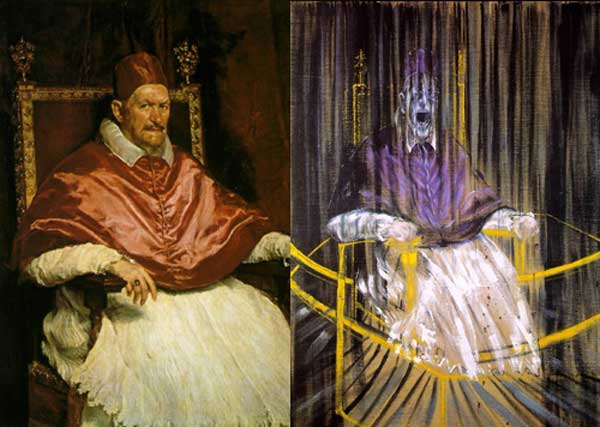The general consensus here was that in going from $\mathbb{Z}$ to $\mathbb{F}_1$ one looses the additive structure and retains only the multiplicative one. Hence, ‘commutative algebras’ over $\mathbb{F}_1$ are (commutative) monoids, and mimicking Grothendieck’s functor of points approach to algebraic geometry, a scheme over $\mathbb{F}_1$ would then correspond to a functor

$h_Z~:~\mathbf{monoids} \longrightarrow \mathbf{sets}$

Such functors are described largely by combinatorial data (see for example the recent blueprint-paper by Oliver Lorscheid), and, if the story would stop here, any Rothko painting could be used as illustration.

Most of the former approaches add something though (buzzwords include ‘Arakelov’, ‘completion at $\infty$’, ‘real place’ etc.) in order to connect the virtual geometric object over $\mathbb{F}_1$ with existing real, complex or integral schemes. For example, one can make the virtual object visible via an evaluation map $h_Z \rightarrow h_X$ which is a natural transformation, where $X$ is a complex variety with its usual functor of points $h_X$ and to connect both we associate to a monoid $M$ its complex monoid-algebra $\mathbb{C} M$. An integral scheme $Y$ can then be said to be ‘defined over $\mathbb{F}_1$’, if $h_Z$ becomes a subfunctor of its usual functor of points $h_Y$ (again, assigning to a monoid its integral monoid algebra $\mathbb{Z} M$) and $Y$ is the ‘best’ integral scheme approximation of the complex evaluation map.

To illustrate this, consider the painting Study after Velázquez’s Portrait of Pope Innocent X by Francis Bacon (right-hand painting above) which is a distorded version of the left-hand painting Portrait of Innocent X by Diego Velázquez.

Here, Velázquez’ painting plays the role of the complex variety which makes the combinatorial gadget $h_Z$ visible, and, Bacon’s painting depicts the integral scheme, build up from this combinatorial data, which approximates the evaluation map best.

All of the former approaches more or less give the same very small list of integral schemes defined over $\mathbb{F}_1$, none of them motivically interesting.

The current approach : Jackson Pollock “No. 8” (1949)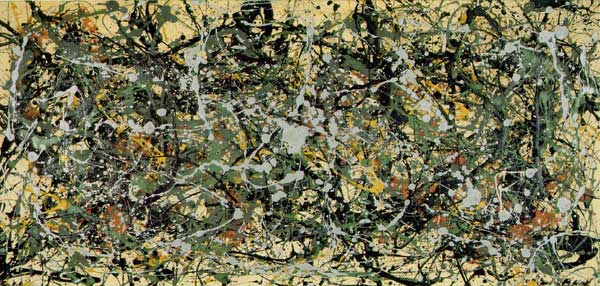An entirely different approach was proposed by James Borger in $\Lambda$-rings and the field with one element. He proposes another definition for commutative $\mathbb{F}_1$-algebras, namely $\lambda$-rings (in the sense of Grothendieck’s Riemann-Roch) and he argues that the $\lambda$-ring structure (which amounts in the sensible cases to a family of endomorphisms of the integral ring lifting the Frobenius morphisms) can be viewed as descent data from $\mathbb{Z}$ to $\mathbb{F}_1$.

The list of integral schemes of finite type with a $\lambda$-structure coincides roughly with the list of integral schemes defined over $\mathbb{F}_1$ in the other approaches, but Borger’s theory really shines in that it proposes long sought for mystery-objects such as $\mathbf{spec}(\mathbb{Z}) \times_{\mathbf{spec}(\mathbb{F}_1)} \mathbf{spec}(\mathbb{Z})$. If one accepts Borger’s premise, then this object should be the geometric object corresponding to the Witt-ring $W(\mathbb{Z})$. Recall that the role of Witt-rings in $\mathbb{F}_1$-geometry was anticipated by Manin in Cyclotomy and analytic geometry over $\mathbb{F}_1$.

But, Witt-rings and their associated Witt-spaces are huge objects, so one needs to extend arithmetic geometry drastically to include such ‘integral schemes of infinite type’. Borger has made a couple of steps in this direction in The basic geometry of Witt vectors, II: Spaces.

To depict these new infinite dimensional geometric objects I’ve chosen for Jackson Pollock‘s painting No. 8. It is no coincidence that Pollock-paintings also appeared in the depiction of noncommutative spaces. In fact, Matilde Marcolli has made the connection between $\lambda$-rings and noncommutative geometry in Cyclotomy and endomotives by showing that the Bost-Connes endomotives are universal for $\lambda$-rings.

One of the more surprising analogies around is that prime numbers can be viewed as knots in the 3-sphere $S^3$. The motivation behind it is that the (etale) fundamental group of $\pmb{spec}(\mathbb{Z}/(p))$ is equal to (the completion) of the fundamental group of a circle $S^1$ and that the embedding

$\pmb{spec}(\mathbb{Z}/(p)) \subset \pmb{spec}(\mathbb{Z})$

embeds this circle as a knot in a 3-dimensional simply connected manifold which, after Perelman, has to be $S^3$. For more see the what is the knot associated to a prime?-post.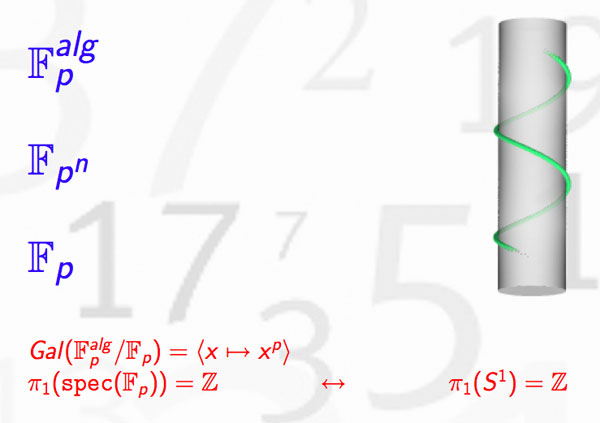In recent months new evidence has come to light allowing us to settle the genesis of this marvelous idea.

1. The former consensus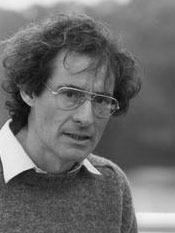Until now, the generally accepted view (see for example the ‘Mazur-dictionary-post’ or Morishita’s expository paper) was that the analogy between knots and primes was first pointed out by Barry Mazur in the middle of the 1960’s when preparing for his lectures at the Summer Conference on Algebraic Geometry, at Bowdoin, in 1966. The lecture notes where later published in 1973 in the Annales of the ENS as ‘Notes on etale cohomology of number fields’.

For further use in this series of posts, please note the acknowledgement at the bottom of the first page, reproduced below : “It gives me pleasure to thank J.-P. Serre for his vigorous editing and his suggestions and corrections, which led to this revised version.”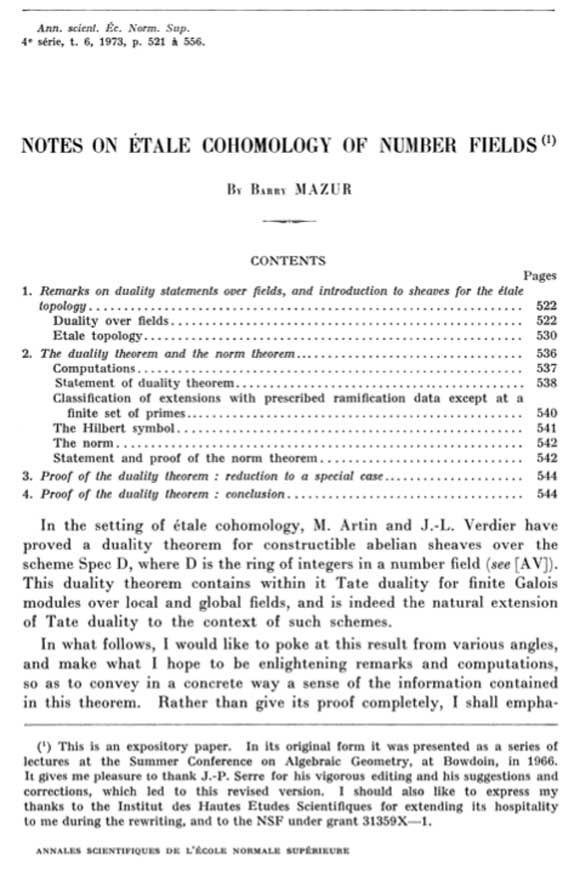Independently, Yuri I. Manin spotted the same analogy at around the same time. However, this point of view was quickly forgotten in favor of the more classical one of viewing number fields as analogous to algebraic function fields of one variable. Subsequently, in the mid 1990’s Mikhail Kapranov and Alexander Reznikov took up the analogy between number fields and 3-manifolds again, and called the resulting study arithmetic topology.

2. The new evidence

On december 13th 2010, David Feldman posted a MathOverflow-question Mazur’s unpublished manuscript on primes and knots?. He wrote : “The story of the analogy between knots and primes, which now has a literature, started with an unpublished note by Barry Mazur. I’m not absolutely sure this is the one I mean, but in his paper, Analogies between group actions on 3-manifolds and number fields, Adam Sikora cites B. Mazur, Remarks on the Alexander polynomial, unpublished notes.

Two months later, on february 15th David Feldman suddenly found the missing preprint in his mail-box and made it available. The preprint is now also available from Barry Mazur’s website. Mazur adds the following comment :

“In 1963 or 1964 I wrote an article Remarks on the Alexander Polynomial [PDF] about the analogy between knots in the three-dimensional sphere and prime numbers (and, correspondingly, the relationship between the Alexander polynomial and Iwasawa Theory). I distributed some copies of my article but never published it, and I misplaced my own copy. In subsequent years I have had many requests for my article and would often try to search through my files to find it, but never did. A few weeks ago Minh-Tri Do asked me for my article, and when I said I had none, he very kindly went on the web and magically found a scanned copy of it. I’m extremely grateful to Minh-Tri Do for his efforts (and many thanks, too, to David Feldman who provided the lead).”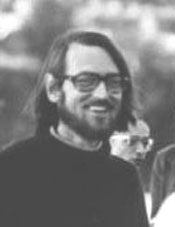The opening paragraph of this unpublished preprint contains a major surprise!

Mazur points to David Mumford as the originator of the ‘primes-are-knots’ idea : “Mumford has suggested a most elegant model as a geometric interpretation of the above situation : $\pmb{spec}(\mathbb{Z}/p\mathbb{Z})$ is like a one-dimensional knot in $\pmb{spec}(\mathbb{Z})$ which is like a simply connected three-manifold.”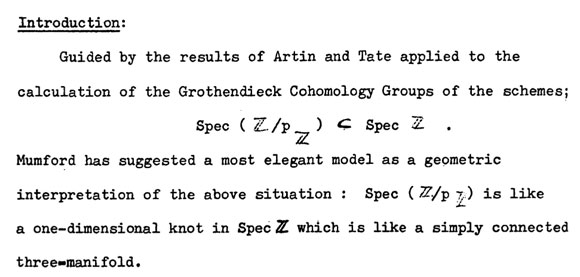In a later post we will show that one can even pinpoint the time and place when and where this analogy was first dreamed-up to within a few days and a couple of miles.

For the impatient among you, have a sneak preview of the cradle of birth of the primes=knots idea…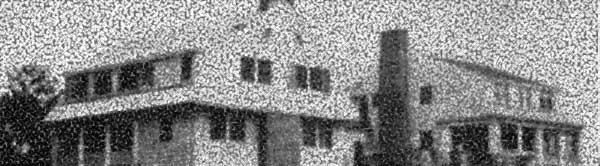Last time we did recall Manin’s comparisons between some approaches to geometry over the absolute point $\pmb{spec}(\mathbb{F}_1)$ and trends in the history of art.

In the comments to that post, Javier Lopez-Pena wrote that he and Oliver Lorscheid briefly contemplated the idea of extending Manin’s artsy-dictionary to all approaches they did draw on their Map of $\mathbb{F}_1$-land.

So this time, we will include here Javier’s and Oliver’s insights on the colored pieces below in their map : CC=Connes-Consani, Generalized torified schemes=Lopez Pena-Lorscheid, Generalized schemes with 0=Durov and, this time, $\Lambda$=Manin-Marcolli.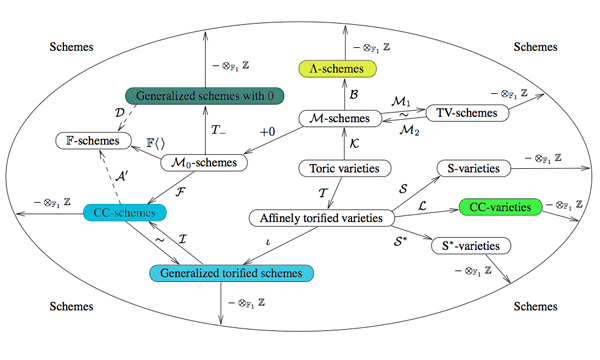Durov : romanticism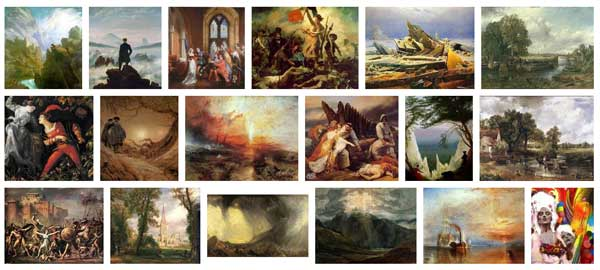In his 568 page long Ph.D. thesis New Approach to Arakelov Geometry Nikolai Durov introduces a vast generalization of classical algebraic geometry in which both Arakelov geometry and a more exotic geometry over $\mathbb{F}_1$ fit naturally. Because there were great hopes and expectations it would lead to a big extension of algebraic geometry, Javier and Oliver associate this approach to romantism. From wikipedia : “The modern sense of a romantic character may be expressed in Byronic ideals of a gifted, perhaps misunderstood loner, creatively following the dictates of his inspiration rather than the standard ways of contemporary society.”

Manin and Marcolli : impressionism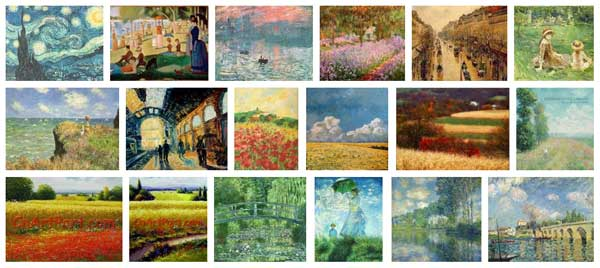Yuri I. Manin in Cyclotomy and analytic geometry over $\mathbb{F}_1$ and Matilde Marcolli in Cyclotomy and endomotives develop a theory of analytic geometry over $\mathbb{F}_1$ based on analytic functions ‘leaking out of roots of unity’. Javier and Oliver depict such functions as ‘thin, but visible brush strokes at roots of 1’ and therefore associate this approach to impressionism. Frow wikipedia : ‘Characteristics of Impressionist paintings include: relatively small, thin, yet visible brush strokes; open composition; emphasis on accurate depiction of light in its changing qualities (often accentuating the effects of the passage of time); common, ordinary subject matter; the inclusion of movement as a crucial element of human perception and experience; and unusual visual angles.’

Connes and Consani : cubism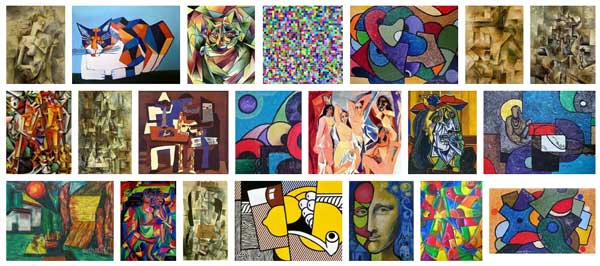In On the notion of geometry over $\mathbb{F}_1$ Alain Connes and Katia Consani develop their extension of Soule’s approach. A while ago I’ve done a couple of posts on this here, here and here. Javier and Oliver associate this approach to cubism (a.o. Pablo Picasso and Georges Braque) because of the weird juxtapositions of the simple monoidal pieces in this approach.

Lopez-Pena and Lorscheid : deconstructivism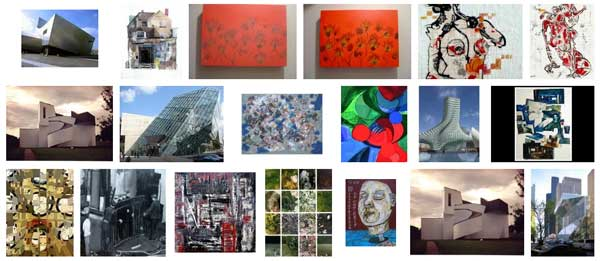Torified varieties and schemes were introduced by Javier Lopez-Pena and Oliver Lorscheid in Torified varieties and their geometries over $\mathbb{F}_1$ to get lots of examples of varieties over the absolute point in the sense of both Soule and Connes-Consani. Because they were fragmenting schemes into their “fundamental pieces” they associate their approach to deconstructivism.

Another time I’ll sketch my own arty-farty take on all this.In his paper Cyclotomy and analytic geometry over $\mathbb{F}_1$ Yuri I. Manin sketches and compares four approaches to the definition of a geometry over $\mathbb{F}_1$, the elusive field with one element.

He writes : “Preparing a colloquium talk in Paris, I have succumbed to the temptation to associate them with some dominant trends in the history of art.”

Remember that the search for the absolute point $\pmb{spec}(\mathbb{F}_1)$ originates from the observation that $\pmb{spec}(\mathbb{Z})$, the set of all prime numbers together with $0$, is too large to serve as the terminal object in Grothendieck’s theory of commutative schemes. The last couple of years have seen a booming industry of proposals, to the extent that Javier Lopez Pena and Oliver Lorscheid decided they had to draw a map of $\mathbb{F}_1$-land.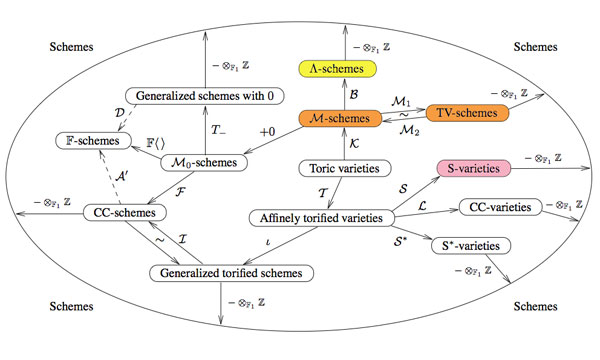Manin only discusses the colored proposals (TV=Toen-Vaquie, M=Deitmar, S=Soule and $\Lambda$=Borger) and compares them to these art-history trends.

Toen and Vaquie : Abstract ExpressionismIn Under $\pmb{spec}(\mathbb{Z})$ Bertrand Toen and Michel Vaquie argue that geometry over $\mathbb{F}_1$ is a special case of algebraic geometry over a symmetric monoidal category, taking the simplest example namely sets and direct products. Probably because of its richness and abstract nature, Manin associates this approach to Abstract Expressionism (a.o. Karel Appel, Jackson Pollock, Mark Rothko, Willem de Kooning).

Deitmar : Minimalism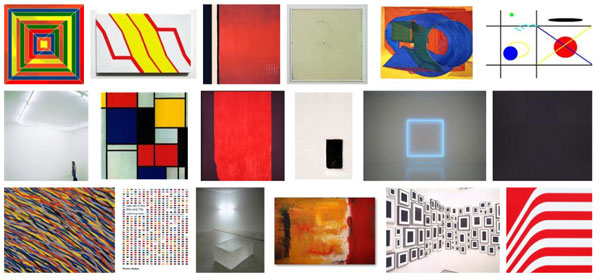Because monoids are the ‘commutative algebras’ in sets with direct products, an equivalent proposal is that of Anton Deitmar in Schemes over $\mathbb{F}_1$ in which the basic affine building blocks are spectra of monoids, topological spaces whose points are submonoids satisfying a primeness property. Because Deitmar himself calls this approach a ‘minimalistic’ one it is only natural to associate to it Minimalism where the work is stripped down to its most fundamental features. Prominent artists associated with this movement include Donald Judd, John McLaughlin, Agnes Martin, Dan Flavin, Robert Morris, Anne Truitt, and Frank Stella.

Soule : Critical Realism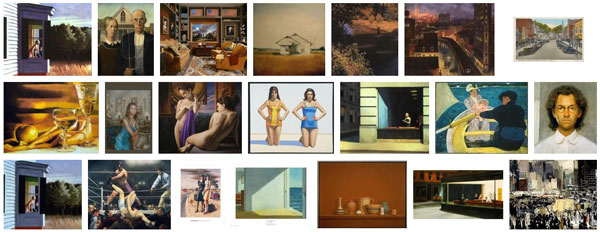in Les varietes sur le corps a un element Christophe Soule defines varieties over $\mathbb{F}_1$ to be specific schemes $X$ over $\mathbb{Z}$ together with a form of ‘descent data’ as well as an additional $\mathbb{C}$-algebra, morally the algebra of functions on the real place. Because of this Manin associates to it Critical Realism in philosophy. There are also ‘realism’ movements in art such as American Realism (o.a. Edward Hopper and John Sloan).

Borger : FuturismJames Borger’s paper Lambda-rings and the field with one element offers a totally new conception of the descent data from $\mathbb{Z}$ to $\mathbb{F}_1$, namely that of a $\lambda$-ring in the sense of Grothendieck. Because Manin expects this approach to lead to progress in the field, he connects it to Futurism, an artistic and social movement that originated in Italy in the early 20th century.

This is a belated response to a Math-Overflow exchange between Thomas Riepe and Chandan Singh Dalawat asking for a possible connection between Connes’ noncommutative geometry approach to the Riemann hypothesis and the Langlands program.

Here’s the punchline : a large chunk of the Connes-Marcolli book Noncommutative Geometry, Quantum Fields and Motives can be read as an exploration of the noncommutative boundary to the Langlands program (at least for $GL_1$ and $GL_2$ over the rationals $\mathbb{Q}$).

Recall that Langlands for $GL_1$ over the rationals is the correspondence, given by the Artin reciprocity law, between on the one hand the abelianized absolute Galois group

$Gal(\overline{\mathbb{Q}}/\mathbb{Q})^{ab} = Gal(\mathbb{Q}(\mu_{\infty})/\mathbb{Q}) \simeq \hat{\mathbb{Z}}^*$

and on the other hand the connected components of the idele classes

$\mathbb{A}^{\ast}_{\mathbb{Q}}/\mathbb{Q}^{\ast} = \mathbb{R}^{\ast}_{+} \times \hat{\mathbb{Z}}^{\ast}$

The locally compact Abelian group of idele classes can be viewed as the nice locus of the horrible quotient space of adele classes $\mathbb{A}_{\mathbb{Q}}/\mathbb{Q}^{\ast}$. There is a well-defined map

$\mathbb{A}_{\mathbb{Q}}’/\mathbb{Q}^{\ast} \rightarrow \mathbb{R}_{+} \qquad (x_{\infty},x_2,x_3,\ldots) \mapsto | x_{\infty} | \prod | x_p |_p$

from the subset $\mathbb{A}_{\mathbb{Q}}’$ consisting of adeles of which almost all terms belong to $\mathbb{Z}_p^{\ast}$. The inverse image of this map over $\mathbb{R}_+^{\ast}$ are precisely the idele classes $\mathbb{A}^{\ast}_{\mathbb{Q}}/\mathbb{Q}^{\ast}$. In this way one can view the adele classes as a closure, or ‘compactification’, of the idele classes.

This is somewhat reminiscent of extending the nice action of the modular group on the upper-half plane to its badly behaved action on the boundary as in the Manin-Marcolli cave post.

The topological properties of the fiber over zero, and indeed of the total space of adele classes, are horrible in the sense that the discrete group $\mathbb{Q}^*$ acts ergodically on it, due to the irrationality of $log(p_1)/log(p_2)$ for primes $p_i$. All this is explained well (in the semi-local case, that is using $\mathbb{A}_Q’$ above) in the Connes-Marcolli book (section 2.7).

In much the same spirit as non-free actions of reductive groups on algebraic varieties are best handled using stacks, such ergodic actions are best handled by the tools of noncommutative geometry. That is, one tries to get at the geometry of $\mathbb{A}_{\mathbb{Q}}/\mathbb{Q}^{\ast}$ by studying an associated non-commutative algebra, the skew-ring extension of the group-ring of the adeles by the action of $\mathbb{Q}^*$ on it. This algebra is known to be Morita equivalent to the Bost-Connes algebra which is the algebra featuring in Connes’ approach to the Riemann hypothesis.

It shouldn’t thus come as a major surprise that one is able to recover the other side of the Langlands correspondence, that is the Galois group $Gal(\mathbb{Q}(\mu_{\infty})/\mathbb{Q})$, from the Bost-Connes algebra as the symmetries of certain states.

In a similar vein one can read the Connes-Marcolli $GL_2$-system (section 3.7 of their book) as an exploration of the noncommutative closure of the Langlands-space $GL_2(\mathbb{A}_{\mathbb{Q}})/GL_2(\mathbb{Q})$.

At the moment I’m running a master-seminar noncommutative geometry trying to explain this connection in detail. But, we’re still in the early phases, struggling with the topology of ideles and adeles, reciprocity laws, L-functions and the lot. Still, if someone is interested I might attempt to post some lecture notes here.